Question

# X-ray Diffraction of Cu (FCC) using X-rays of wavelength =1.54Å gives XRD pattern where one of...

X-ray Diffraction of Cu (FCC) using X-rays of wavelength =1.54Å gives XRD pattern where one of the peaks occurs at 2=43.2º What are the Miller indices for this peak? Given atomic radius for Cu is 0.128nm (note a=22 r)

The Miller indices for this peak is ( 111 ).

This problem can be solved by applying Bragg's law.

The detail calculation is shown below.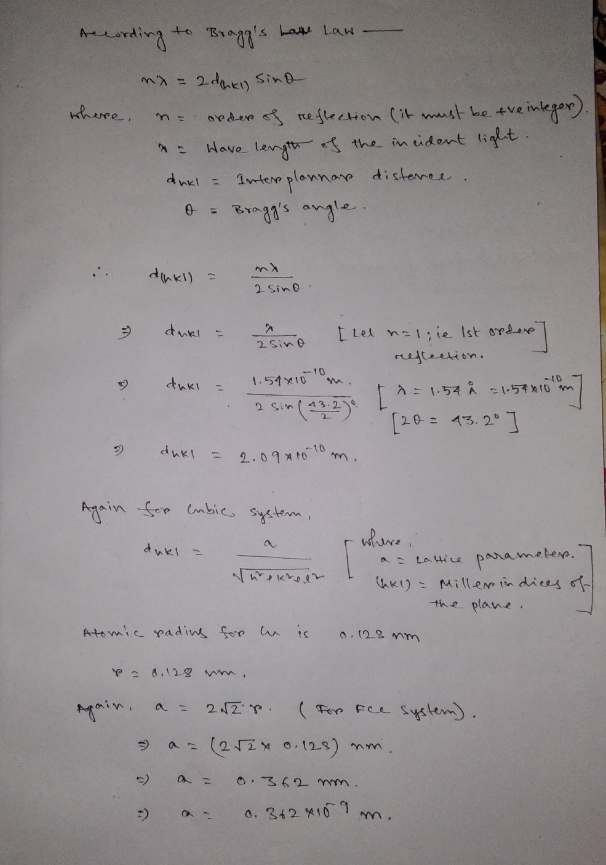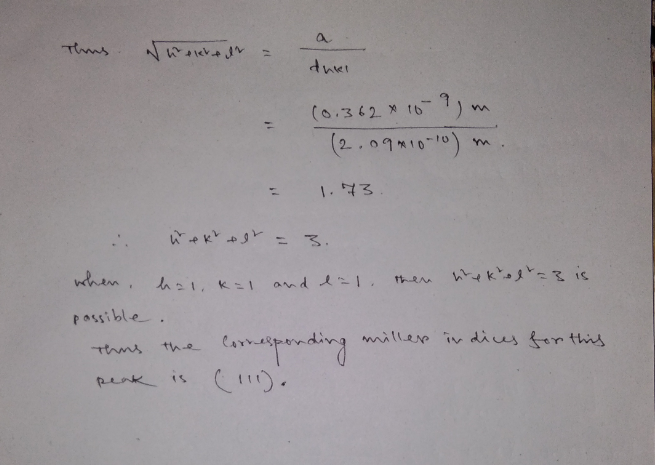#### Earn Coins

Coins can be redeemed for fabulous gifts.

Similar Homework Help Questions
• ### In X-ray diffraction (XRD), not all planes with atoms will create peaks in the XRD pattern,...

In X-ray diffraction (XRD), not all planes with atoms will create peaks in the XRD pattern, True or False? Please provide an explanation.

• ### X-Ray Diffraction (XRD) is a wonderful tool for characterizing biomaterials. Please answer the following questions using...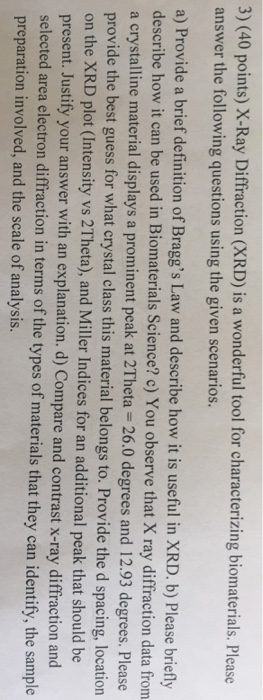X-Ray Diffraction (XRD) is a wonderful tool for characterizing biomaterials. Please answer the following questions using the given scenarios. Provide a brief definition of Bragg's Law and describe how it is useful in XRD. b) Please briefly describe how it can be used in Biomaterials Science? c) You observe that X ray diffraction data from a crystalline material displays a prominent peak at 2Theta = 26.0 degrees and 12.93 degrees. Please provide the best guess for what crystal class this...

• ### 4. Lead (Pb) has an FCC crystal structure. Lead was studied using monochromatic X-rays and the...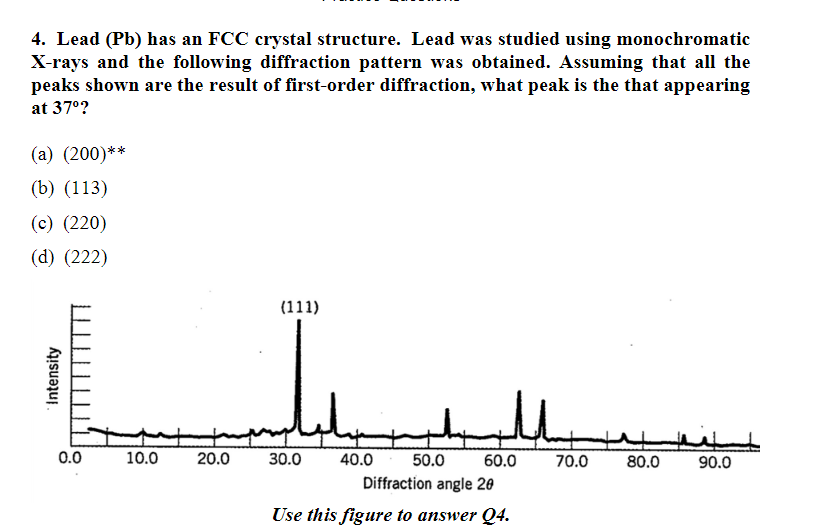4. Lead (Pb) has an FCC crystal structure. Lead was studied using monochromatic X-rays and the following diffraction pattern was obtained. Assuming that all the peaks shown are the result of first-order diffraction, what peak is the that appearing at 370? (a) (200)** (b) (113) (c) (220) (d) (222) (111) 0.0 10.0 20.0 30.0 40.0 50.0 60.0 80.0 70.0 90.0 Diffraction angle 20 Use this figure to answer Q4 Intensity 4. Lead (Pb) has an FCC crystal structure. Lead was...

• ### 3-77 Figure 3-43 shows the results of an X-ray diffrac- tion experiment in the form of...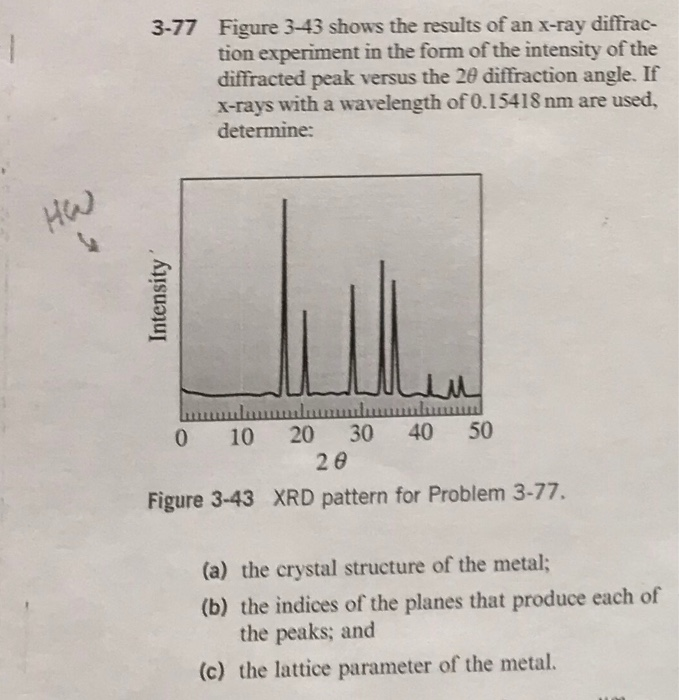3-77 Figure 3-43 shows the results of an X-ray diffrac- tion experiment in the form of the intensity of the diffracted peak versus the 20 diffraction angle. If X-rays with a wavelength of 0.15418 nm are used, determine: Intensity ULLA mmmmmmmmmmmmm 0 10 20 30 40 50 28 Figure 3-43 XRD pattern for Problem 3-77. (a) the crystal structure of the metal; (b) the indices of the planes that produce each of the peaks; and (c) the lattice parameter of...

• ### An X-ray diffraction analysis of crystalline CsCl using X-rays of wavelength 86.1 pm has a prominent peak at 2θ = 19.1°....

An X-ray diffraction analysis of crystalline CsCl using X-rays of wavelength 86.1 pm has a prominent peak at 2θ = 19.1°. If this peak corresponds to n = 2, what is the spacing between the ion layers?

• ### Q1- The atoms in a crystal are modelled as hard spheres in contact. Calculate the Atomic radius i...

Q1- The atoms in a crystal are modelled as hard spheres in contact. Calculate the Atomic radius in a fcc lattice with lattice constant 3.6 A Q2- (a) The atomic in a crystal are modelled as hard spheres in constant. Calculate the atomic radius in a fcc lattice with lattice constant 3.6 A. (b) Consider three-dimensional simple cubic lattice with lattice constant a. What is the distance from the center to a corner of the first Brillouin Zone in the...

• ### Characteristic x-rays: 30 keV electons emitted X-ray spectrum (shown below) is measured. are inci...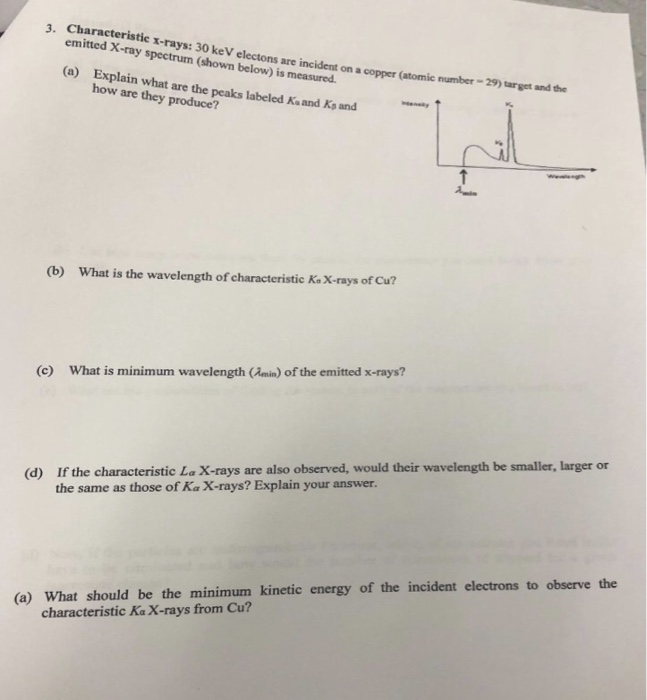Characteristic x-rays: 30 keV electons emitted X-ray spectrum (shown below) is measured. are incident on a copper (atomic number- 29) target and the (a) Explain what are the how are they produce? peaks labeled Ka and Kp and What is the wavelength of characteristic Ks X-rays of Cu? (b) What is minimum wavelength (Jmin) of the emitted x-rays? (c) If the characteristic La X-rays are also the same as those of Ka X-rays? Explain your answer. observed, would their wavelength...

• ### Problem 2: X-ray diffraction: (a) Determine the maximum wavelength for which constructive interference can be observed...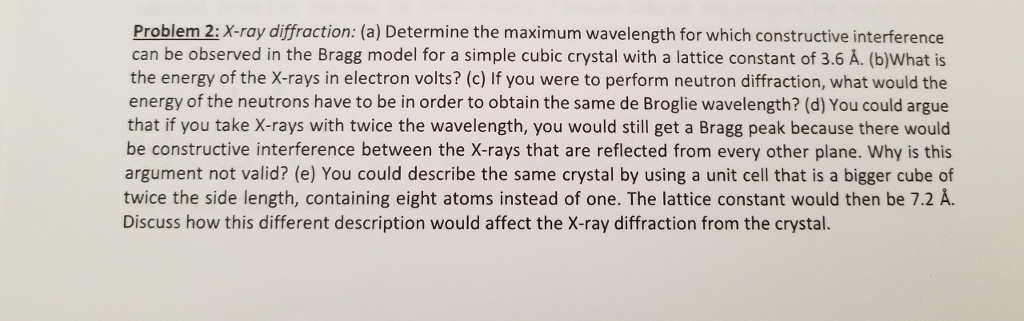Problem 2: X-ray diffraction: (a) Determine the maximum wavelength for which constructive interference can be observed in the Bragg model for a simple cubic crystal with a lattice constant of 3.6 A. (b)What is the energy of the X-rays in electron volts? (c) If you were to perform neutron diffraction, what would the energy of the neutrons have to be in order to obtain the same de Broglie wavelength? (d) You could argue that if you take X-rays with twice...

• ### Item 3.5 X-rays with a wavelength of 0.0711 nm create a diffraction pattern when they pass...

Item 3.5 X-rays with a wavelength of 0.0711 nm create a diffraction pattern when they pass through a protein crystal. Part A If the angular spacing between adjacent bright spots (m=0 and m=1) in the diffraction pattern is 35.9 ∘, what is the average spacing between atoms in the crystal?

• ### Suppose you want to produce a diffraction pattern with X-rays whose wavelength is 0.030 nm ....

Suppose you want to produce a diffraction pattern with X-rays whose wavelength is 0.030 nm . Part A If you use a diffraction grating, what separation between lines is needed to generate a pattern with the first maximum at an angle of 15 ∘ ? (For comparison, a typical atom is a few tenths of a nanometer in diameter.) ??? nm